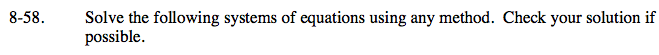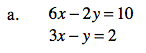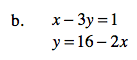### Home > CAAC > Chapter 8 > Lesson 8.2.2 > Problem8-58

8-58.You can use the elimination method.

Multiply the second equation by −2.

Add the two equations together, combining like terms.

There is no solution.

−2(3xy = 2)
−6x + 2y = − 4

6x − 2y = 10
−6x + 2y = −4
0 = 6You can use the substitution method.

Distribute and solve for x.

Combine like terms.